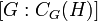# FC-group

This article defines a group property: a property that can be evaluated to true/false for any given group, invariant under isomorphism
View a complete list of group properties
VIEW RELATED: Group property implications | Group property non-implications |Group metaproperty satisfactions | Group metaproperty dissatisfactions | Group property satisfactions | Group property dissatisfactions

## Definition

### Equivalent definitions in tabular format

No. Shorthand A group is termed a FC-group if ... A group$G$ is termed a FC-group if ...
1 conjugacy classes are finite every conjugacy class in it has finite size. for every$x \in G$, the conjugacy class of$x$ in$G$ is finite.
2 element centralizers have finite index the centralizer of any element is a subgroup of finite index. for any$x \in G$, the index$[G:C_G(x)]$ of the centralizer$C_G(x)$ is finite.
3 finite subset centralizers have finite index the centralizer of any finite subset is a subgroup of finite index. for any finite subset$S \subseteq G$, the index$[G:C_G(S)]$ of the centralizer$C_G(S)$ is finite.
4 finitely generated subgroup centralizers have finite index the centralizer of any subgroup generated by a finite subset is of finite index. for any finitely generated subgroup$H$ of$G$, the index$[G:C_G(H)]$ is finite.

## Metaproperties

Metaproperty name Satisfied? Proof Statement with symbols
subgroup-closed group property Yes Suppose$G$ is a FC-group and$H$ is a subgroup of$G$. Then,$H$ is also a FC-group.
quotient-closed group property Yes Suppose$G$ is a FC-group and$H$ is a normal subgroup of$G$. Then, the quotient group$G/H$ is a FC-group.
finite direct product-closed group property Yes Suppose$G_1$ and$G_2$ are FC-groups. Then, so is the external direct product$G_1 \times G_2$.

## Relation with other properties

### Stronger properties

Property Meaning Proof of implication Proof of strictness (reverse implication failure) Intermediate notions
finite group has only finitely many elements obvious any infinite abelian group works as a counterexample. FZ-group, Finitely generated FZ-group, Group with finite derived subgroup|FULL LIST, MORE INFO
abelian group all conjugacy classes have size one obvious any finite non-abelian group works as a counterexample. FZ-group, Group with finite derived subgroup|FULL LIST, MORE INFO
FZ-group the center has finite index FZ implies FC FC not implies FZ Group with finite derived subgroup|FULL LIST, MORE INFO
group with finite derived subgroup the derived subgroup is finite finite derived subgroup implies FC FC not implies finite derived subgroup |FULL LIST, MORE INFO
BFC-group there is a common bound on the sizes of all conjugacy classes FC not implies BFC |FULL LIST, MORE INFO

## Study of this notion

### Mathematical subject classification

Under the Mathematical subject classification, the study of this notion comes under the class: 20F24

The subject classification 20F24 is used for FC-groups, and their generalizations.